You are here : Main projects | The Zeus Tesla Coil | 3. Conception and construction

The Zeus Tesla Coil
September 2011

# 3.  Conception and construction

This chapter deals with the formulas used to conceive the Zeus Tesla coil and also with specific details of practical scope.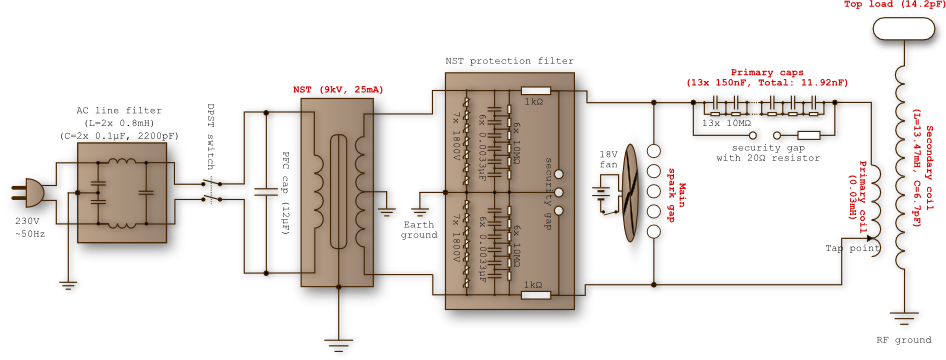Figure 3.1 — Complete electrical schematic of Zeus. Core components are annoted in red.

Important remark. Building/designing a Tesla coil is a far less tedious task with adequate programs that perform the necessary computations. I recommend both Bart Anderson's JAVATC and Kevin Wilson's TeslaMap for theses purposes, as they have proven instrumental in the construction of Zeus.

## 3.1   HV transformer

The high voltage transformer is the most important part of a Tesla coil. It is simply an induction transformer. Its role is to charge the primary capacitor at the beginning of each cycle. Apart from its power, its ruggedness is very important as it must withstand terrific operation conditions (a protection filter is sometimes necessary, see below).

Among the most common are the pole pigs, normally used in the electrical grid. These typically provide around 20kV and have no integrated current limitation (large ballasts inductors are required to limit the current), which makes them quite deadly if not handled correctly. Obtaining one is quite difficult in Europe. The other widely-used type of transformer is the neon sign transformer (NST), which is, as its name suggests, generally used to power neon signs. They generally supply between 6 and 15 kV and are current-limited often at 30 or 60 mA. They are safer and easier to find than the pole pigs but are more fragile. Notice that newer NST should be avoided, as they are provided with a built-in differential circuit breaker, which will prevent any Tesla coil operation. Indeed, we'll later see that Tesla coil operation provokes repeated spikes of current and voltages that will trigger the breaker. Beside from these two common types, one can also use a flyback transformer or a microwave oven transformer (MOT).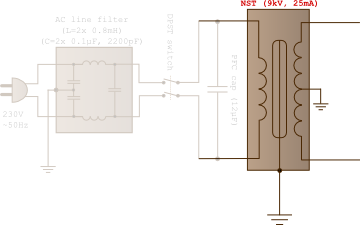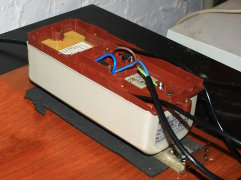Figure 3.2 — Position on schematic (highlighted) and photograph of Zeus' NST (out of his wooden box).

The power supply of Zeus consists of a single NST, whose characteristics (rms values) are the following :

Voltage = 9000 V
Current = 25 mA

We can now compute its power P = VI, which will be useful to set the global dimensions of the Tesla coil as well as a rough idea of its sparks' length.

P = 225 W

Zeus has thus a relatively low power, which is perhaps not a bad thing for a very first coil. For more power, one can connect several transformers in parallel in order to increase output current. We'll also need to know the transformer's impedance in order to compute the optimal size of the primary capacitor. Using Ohm's law Z = V/I, one finds :

Z = 360 kΩ

### 3.1.1   Arc length

There's an empirical formula giving the maximal length of the sparks only from the output power of the transformer. For the reasons mentioned in the energy losses subsection (previous page), it's very difficult to reach this value (but a few expert have however surpassed it). The phenomenon of gas breakdown is extremely complex, and the arc length depends on much more than simply the voltage at the top load. The basic formula giving the length L of the sparks in centimetres is the following :

L ≈ 4.32 (Pin)1/2

where Pin is the power output of the transformer in watts. We apply the formula to Zeus (225 W) :

L ≈ 65 cm

There are however more precise formulas, which notably take into account the Q factor of the secondary coil and the firing frequency of the spark gap. The JAVATC simulation gave the value of 55 cm. My personal record is 45 cm, which is not too bad, given the type of the spark gap used. The arcs generated by Zeus are generally between 30 and 35 cm, with occasionally some arcs longer than 35 cm. My second coil, which has several of its components better designed, has a far better efficiency, achieving similar arc length with only 2/3 of Zeus' power.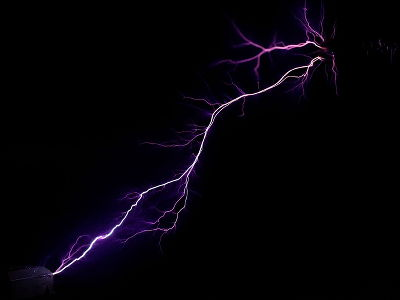Figure 3.3 — A 40 cm spark (looks like a natural one!). 1/30s shutter speed.

## 3.2   Primary circuit

### 3.2.1   Capacitor

The role of the primary capacitor is to store a certain quantity of charge (thus of energy) for the coming cycle, as well as forming an LC circuit along with the primary inductor. This is certainly the most difficult component to make on one's own, I had to rebuilt it three time before getting a working and efficient version. The primary capacitor is indeed subject to very rough treatment : it must withstand strong spikes of current (hundreds of amps) and voltage as well as very short charge/discharge times, and must at the same time boast low dielectric losses at radio frequencies. For performance-related issues, I finally switched to factory capacitors.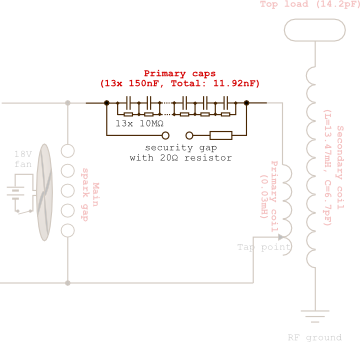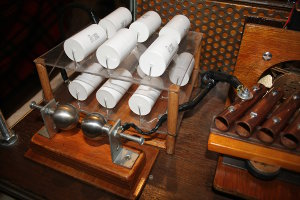Figure 3.4 — Position on schematic (highlighted) and photograph of Zeus' latest working capacitor.

Its capacitance must be such as there is resonant amplification in the primary circuit (remember : we have an LC circuit in series with an alternating voltage generator). Let's call Cres this specific value. If the capacitance is lower than Cres the energy available for the rest of the cycle will be lower. The same thing would happen if the capacitance is larger than Cres, but the larger capacitance allows more charge to be stored, which compensates the first problem. We'll see in resonant charge subsection that it might be judicious to make a "bigger" capacitor in order to prevent the amplification from becoming too powerful, which could easily destroy the transformer as well as the capacitor.

By definition, we could find Cres with the resonant frequency formula for 50 Hz, but we must know the total inductance of the primary circuit. We'll rather use a formula involving the impedance Z. It's important to note that the inductance of the transformer is much greater than the inductance of the primary coil, and the same is true for their impedances ; we'll therefore neglect the primary inductor's contribution. The aforementioned formula is the following:

Cres = (2πZf)-1

The mains current is 50 Hz in Europe and the impedance of our NST is 3.6·105 Ω. We thus immediately get :

Cres = 8.84 nF

For the reason mentioned above and because of practical constrains, I didn't respect this value when I built Zeus' capacitor. Its (measured) capacity is actually :

C = (11.92 ± 0.01) nF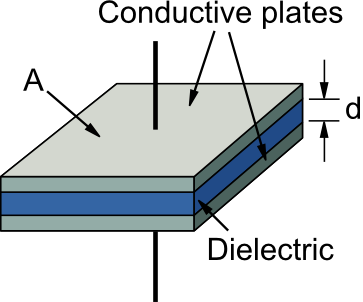Building your own capacitor is a enriching experience but you should keep in mind that its performances will inevitably be lower than those of factory-made capacitors. A basic design capacitor of this kind is shown on the right.

Using Maxwell's equations, it's possible to find a simple formula giving the capacitance C of a basic capacitor, made of two plate of area A separated by a distance d by a dielectric of permittivity ε. One can show that

C = εA/d

To increase capacitance, one can put several plates in parallel instead of just two. Then, half of the plates are, say, positive while the other half are negative. If this capacitor is made of n plates, there is (n-1) capacitors in parallel, and its capacitance is thus:

C = (n-1)εA/d

The choice of the dielectric medium is of prime importance. It must have a high dielectric strength and provide low losses at high frequencies. The dielectric strength is the minimum electric field required to provoke a breakdown of the medium. The dielectric losses come from the fact that a real capacitor also possess a resistive component to its impedance (see fig. 4.6). A fraction of the energy is then lost in the form of heat in the dielectric medium and in the conductors. (Please note that the former is typical to alternating current. When a capacitor is charged with direct current, no such losses occur : all the energy is recovered at discharge.) It is however the dielectric losses that predominates and a loss tangent is defined for each dielectric.

Figure 3.5 — A real capacitor has an internal resistance in addition to its impedance, which is equivalent to series resistance (ESR). [Images credits : Wikipedia]

The resistive component of the capacitors impedance is called equivalent series resistance (ESR) and is measured by connecting a resistor in parallel to capacitor through this formula (more details on the Wikipedia article) :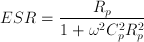where Rp is the resistance of the parallel resistor, ω the pulsation of the alternating current and Cp the capacitance of the ideal capacitor. The tangent loss is defined as the ratio of the real to imaginary part of the capacitor's impedance (notice the analogy with the Q factor of an oscillator) :

tan(δ) = ESR/Xc

Here is a list of possible dielectrics for high-voltage applications (references from which this table has been compiled can be found on the PDF version). We can see that the LDPE (low density polyethylene) is a good dielectric for making Tesla coil capacitors, as it has all the required qualities and is easy to find.

Dielectric medium εr at 1 MHz Dielectric strength [kV/mm] tan(δ) at 1 MHz
LDPE 2.25 18.9 - 26.7 8·10-5
PP 2.20 - 2.36 24 2·10-4
PVC 3.00 - 3.30 18 - 50 0.015 - 0.033
Glass 4.7 17.7 0.038
Neoprene 6.26 15.7 - 26.7 0.038

Finally, when dealing with high voltages, there's certain principles to respect in order to built a enduring cap :

• A shorter distance between the plates will lead to a higher capacitance, but will increase the risk of breakdown as the field will be more intense. There's some sort of minimal distance to respect. For LDPE, I recommend at least 0,15mm per kV (rms) supplied by the transformer.
• It can be demonstrated that the electrical field surrounding spikes tend to be much more intense (spike effect). Therefore, the risk of breakdown will be higher at the corner of the plates. The corners and edges of the plates must be rounded and the surfaces must be clear of any scratch.
• The dielectric medium is likely to have imperfections (microscopic holes, impurities, etc.), which also increases the risk of breakdown. For a given thickness, it is preferable to use multiple thinner layers instead on a single thick one. If one layer was to be damaged, the others will remain intact.
• Corona effect around the plates will gradually attack the adjacent dielectric layers, which is one more reason to prefer multi-layered dielectric. The best protection for a HV capacitor is to be drowned in an insulating oil like transformer oil (it's purified mineral oil -like silicon oil-, which has the advantage of not carbonizing). For a given capacitance, it is also better to build several larger capacitors with thinner dielectric and to connect them in series. This will distribute the voltage on all the sub-capacitors and thus attenuate the Corona effect, which increases the whole capacitor's survivability for a given voltage. It is recommended to put no more than 5 kV (rms) per sub-capacitor.

I made three attempts before having a working, Tesla coil operation-worthy capacitor. Here are the successive versions.

1st version.   This very first capacitor was made of aluminium plates placed in an alternating pattern (see fig. 3.8). Plates of same polarity were connected with an external screw thread that passed through holes drilled near the edge. The dielectric material was PVC. However, the capacitance of this cap was far lower than the predicted value. I think this was because the holes were too wide to allow a good connection. I never used it on Zeus, but instead reused the plates to built a new, improved capacitor.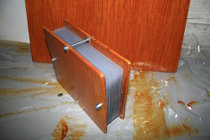Figure 3.6 — v.1, never used.

2nd version.   This second prototype has the same building pattern as the first, i.e. alternating plates. The major difference with the first version is the dielectric medium, which is now LDPE, much more adapted than PVC for Tesla coil operation (greater dielectric strength and reduced losses at radio frequencies). Moreover, the supports were changed to PVC instead of wood, which allowed for better compression of the stack.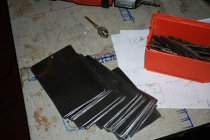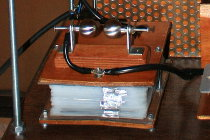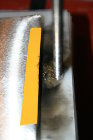Figure 3.7 — v.2 in construction, mounted on Zeus' chassis, and ultimate demise (read below).

The dielectric is made of four sheets of 0.2mm thick LDPE, which gives a 0.8mm spacing between plates. This capacitor should thus resist voltages of at least 11.34 kV. Insulating varnish was also applied to the plates to increase survivability. It was made of 31 plates, whose overlap area was equal to 100 mm × 125 mm = 12500 mm2. Its theoretical capacitance was thus (using the above formula) :

Cth = 9.54 nF

I knew however that construction defects would result in a lower real capacitance. The targeted capacitance was set a bit "too high" in order to attain a real capacitance close to the resonant value, computed previously. Indeed, the overlapping area is slightly inferior to the predicted values because, first, the corners are rounded, and second, the overlapping is not perfect. Moreover, the capacitor has a tendency to "inflate" because of the light weight of the LDPE sheets, and the compression with the two PVC plates was imperfect also. Finally, its measured capacitance was

Cexp = (8.31 ± 0.01) nF

To connect the plates, I had first used aluminium tape (visible in Fig 3.7), but this solution would have made sense only if the stack was immersed in oil. These sheets are indeed very thin and creases form easily, which made the surfaces very rough. When I lighted Zeus the first time, sparks were springing everywhere... on this band of aluminium tape because of Corona effect. It eventually broke because of the high currents.

Figure 3.8 — Schematics for the capacitors v.2 (similar to v.1).

Another means of connection was thus to be found, and I reused the design from the first version : a screw thread passing through holes drilled in the plates. This time, the holes were much smaller for the electrical connection to be tight. However, I was doubtful on this conception as the risk of sparks between the plates and the thread (of different polarity) was high, so insulating tape was put on the edges. Doubts were justified : after a few seconds, a bright light appeared in the capacitor with a strong smell of burned plastic : the capacitor was dead.

After several unsuccessful reparations, it became clear a brand new capacitor design was needed.

3rd version.   This design turned out to be the good one. The capacitor is actually formed of two identical capacitors connected in series. As we saw earlier, this distributes the voltages between the two caps. Here, each cap is submitted to a voltage of "only" 4500 V (rms), which considerably diminishes the harmful Corona effect.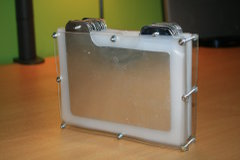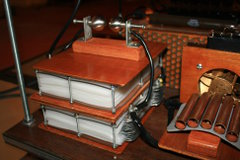Figure 3.10 — Left: An unit of v.3. Right: Both units connected in series and mounted on Zeus' chassis.

The geometry of the plates has changed as well. In order to avoid the problem of arcing between the threads and the plate's edges, a "tab" configuration was used instead (see figs. 3.10 and 3.11), where the connections between plates of same polarity are much more distant of each other. This shape was harder to manufacture, but revealed to be considerably safer. The idea of this configuration came to my mind thanks to Herbert Mehlose's and Jochen Kronjaeger's websites. Each capacitor has 37 plates, whose overlapping area is 100 mm × 150 mm = 15000 mm2.

The relative spacing between the plates has also been increased. On both capacitors, the plates are separated by three sheets of LDPE whose thickness is 0.2mm, for a total of 0.6mm. As the two caps are in series, it is as if the total spacing is 1.2mm. The assembly can therefore resist voltages up to 22.7 kV (rms), nearly indestructible. It is indeed advised that the primary capacitors should be able to withstand 2 to 3 times the rms voltages of the transformer.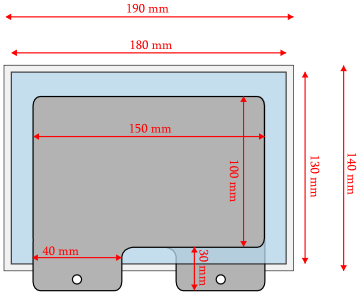Figure 3.11 — Schematic for capacitor v.3 (same color code as in fig. 4.8).

The capacitance formula yields a theoretical value of (per unit)

Cth = 18.32 nF

and the total capacitance of these two identical caps connected in series is thus half the previous value :

Cth, tot = 9.13 nF

Apart form the above modification, the supports were also improved. The PVC plates are attached with 8 screws instead of 4, making the "sandwich" compression much better. Finally, much more insulating varnish was applied. A curious fact was the capacitances of both capacitors seemed to increase slightly day by day. I think this was due to the fact that the plates gradually submitted to the imposed compression. Here are the measured (exp) capacitances:

Cunit 1, exp = (17.78 ± 0.01) nF
Cunit 2, exp = (18.10 ± 0.01) nF

Once both unit were assembled, I measured the total capacitance one more time:

Ctot, exp = (8.96 ± 0.01) nF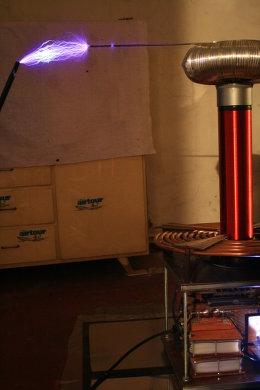Figure 3.12 — This capacitor has indeed driven Zeus, and eventually did not fail. 1s shutter speed.

While Zeus was firing, small flashed could be seen inside the capacitors. These were seemingly not due to dielectric breakdown as the capacitors continued to work. I would say these were due to overheating air bubbles, but without certitude. (ideas?)

#### Cornell-Dubilier 942C capacitors

Despite the "home-made" caps working well, I wanted to build a spare capacitor (just in case), made of industrial caps. When I mounted it on Zeus, it turned out its performance were slightly better, so I decided to keep them as the permanent primary caps. So... 4th version ! High voltage industrial capacitors are much more complex than the basic plate-stack design we just considered, and some additional criteria must be taken into account. K. Wilson has written a list of the qualities a Tesla coil cap should have as well as a list of good caps / bad caps.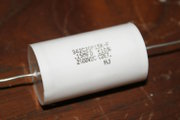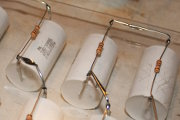Figure 3.13 — Left: An unit of v.4. Right: v.4 in construction.

I used the Cornell Dubiler 942C20P15K-F capacitors because they meet all these requirements (see datasheet) and have been tested and recommended by T. Fritz. These can tolerate up to 2000 V and have a capacitance of 0.15 μF ± 10%. Note that the indicated voltage is the DC tolerance but in a Tesla coil, they'll have to deal with short pulses, so this value can actually be used. By connecting 13 of them in series, one gets

Cexp = (11.92 ± 0.01) nF
Tolerance = 26 000 V

The notion of dV/dt represents the speed at which a capacitor is charged or discharges (its units are V/s). In this context, it's not really the time-derivative of the potential but rather the maximal value it can take. For example, in a sinusoidal signal V(t) = Asin(ωt) like we find in Tesla coils, the dV/dt correspond to the value of the derivative V'(t) = Aωcos(ωt) evaluated at t=2nπ (n integer), i.e. Aω.

The 942C20P15K-F boasts a dV/dt of 2879 V/μs. Let's check if we don't exceed this value in the case of Zeus. The amplitude of the NST's voltage is equal to Vmax = 21/2Vrms = 12 728 V. But with 13 capacitors in series, each one is exposed to only one thirteenth of this value, i.e. 979 V. For the angular speed, we know ω = 2πf where the frequency f is the resonant frequency. We'll later see it is equal to 300 kHz in our case. We thus getWhich is indeed smaller than the value of the datasheet.

Details on construction.   When building such a capacitor, it is very important to use so-called bleeder resistors. These are large ohmic value (generally from 1 to 10 MΩ) resistors connected in parallel to each capacitor (see fig. 4.13). Their role is to ensure a smoother charge/discharge cycle and to prevent the capacitor from holding a dangerous charge. Moreover, they'll allow the capacitors to discharge after the machine has been switched off. I used resistors of 10 MΩ, 0.5 W (see datasheet). It is also recommended to build the capacitor is such a way to avoid arcing between a capacitor and its resistor, they have thus been soldered on opposite sides of the PVC support.

In the same philosophy of extending the caps' life, it is also recommended to put a small resistance of a few Ohms in series with the security spark gap. It must have a high power rating, several hundreds of Watts, and must be non-inductive to avoid the apparition of an extra, parasitic RLC circuit around the primary capacitor. I used a 20 Ohms, 100 Watts, thick film-type resistor (see datasheet), visible on the left extremity of the brown wire on the next picture.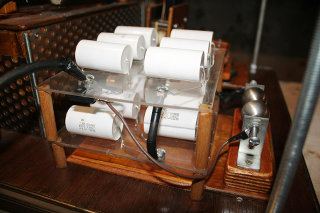Figure π — v.4 mounted on Zeus' chassis.

### 3.2.2   Charge at resonance

Let's talk briefly about the "good" capacitance of the primary capacitor. In the previous section, we had chosen its capacity so a resonant amplification occurs at 50 Hz. This ensures all the energy of the transformer is used, as the impedances of the transformer and the primary circuit are identical. This choice is risky however, as in case of spark gap fault, voltage and current rise to enormous values in a few tenths of a second, a condition which can destroy the caps as well as the transformer. The next plots show how the voltages evolves without (left) and with a static spark gap (right). The latter is a much more realistic description of the evolution inside a Tesla coil. Simulations for a 10 kV, 100 mA NST ; L = 318 H ; R = 5000 Ω.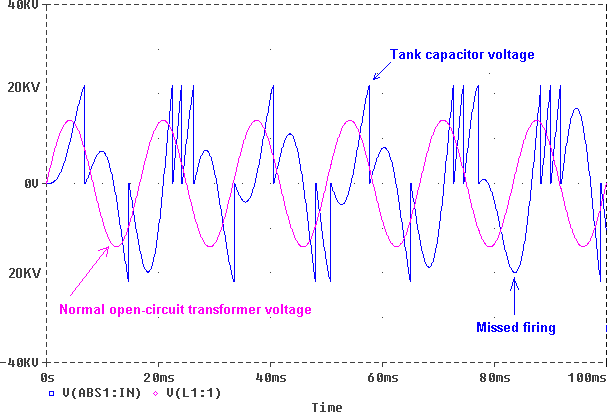Figure 3.15 — Left : Plot of the NST shorted (violet) and in primary circuit (blue) output voltage over time in case of resonance. Right: Plot of the voltage output of the transformer (green) and the voltage across the capacitor (blue) over time with a static spark gap.
[Images credits: R. Burnett]

The next plot show the maximal values that voltage and current can attain in a primary circuit fed by a 10 kV, 100 mA NST in series with a resonant-sized cap. The ballasts' inductance is 318 H and the total resistance is 5000 Ω. We can see that, without a spark gap (or any other device evacuating the energy), voltage can rise up to 200 kV and current up to 2 A.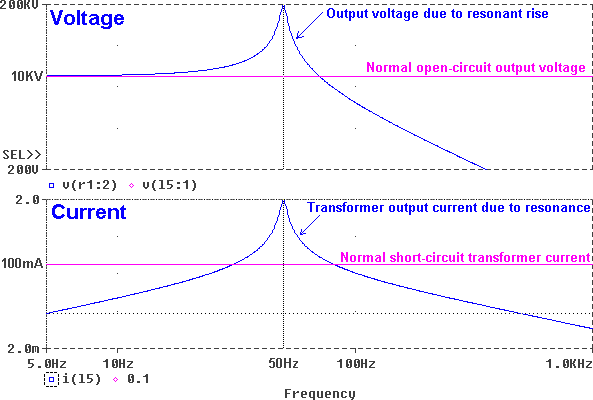Figure 3.16 — Using a resonant-sized capacitor will have the voltage and the current reach values far beyond what components can withstand.
[Image credits: R. Burnett]

Normally, the spark gap will fire above a given voltage (which is far lower than the previous values), making the current and the voltages across the capacitor fall to acceptable levels. But, for various reasons, the spark gap may fail to fire once in a while. This is quite frequent actually, especially for static spark gaps such as Zeus' gap, as they have a tendency to fire rather erratically, as show in fig. 3.15 - Right.

#### Conclusions

The resonant amplification in the primary circuit is a very important phenomenon for good Tesla coil performances, but it must remain under control so as not to damage the components. This is why it is strongly recommended to place security devices around sensitive components. In this philosophy, a security spark gap is generally placed at the lead of the primary capacitor (visible on fig. 4.3). The NST protection filter is a bit more complex and we'll talk about it later.

It is also recommended to use a non-resonant-sized capacitor, in other words, whose capacitance will not lead to a brutal resonant amplification. This larger-than-resonance (LTR) value depends on the type of spark gap used. For a static spark gap, typical values for the LTR capacitance range from 1.5 to 2 times the resonant capacitance (formula given earlier). K. Wilson recommends the value of 1.618 times the resonant value, as this value (an estimation of the golden ratio), will ensure no common integer multiples. Following this approach, the LTR capacitance of Zeus will be around

CLTR = 14.30 nF

The capacitance of Zeus' primary caps is approximately 1.35 times the resonant value, which is perhaps a bit close to the resonant value. But the various protection measures seem to be working well as no sensitive component has been damaged (so far). As mentioned earlier, such an increase in the primary capacity has almost no consequences on the global performances of a Tesla Coil. R. Burnett has shown that, with a static spark gap, the delivered power depends much more on the spark gap spacing than on the primary capacity.

### 3.2.3   Inductor

The role of the primary inductor is to generate a magnetic field to be injected into the secondary circuit as well as forming an LC circuit with the primary capacitor. This component must be able to transport heavy current without excessive losses. The resonance tuning is traditionally made on this component as we'll see later.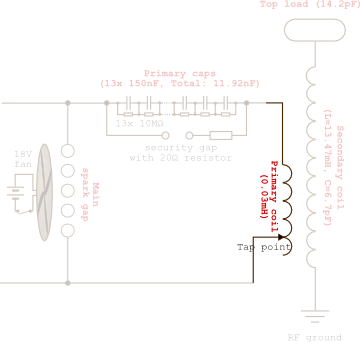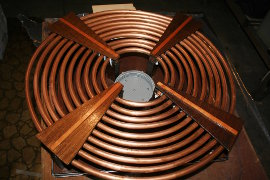Figure 3.17 — Position on schematic (highlighted) and photograph of Zeus' primary inductor (secondary circuit removed).

The primary winding has been tapped to 8.25 turns and has a measured self-inductance of

Lexp = (0.03 ± 0.01) mH

This very approximative value has been measured on a small LCR bridge. A more precise device could be used but the tuning is made via a trial-and-error method, so knowing the exact inductance is not of prime importance. Different geometries are possible for the primary coil. We'll look at the most common one before examining more thoroughly the case of Zeus. Note that the formula giving the self-inductances for each of them are approximative and are just used to have a rough idea of the number of turns required to achieve proper tuning.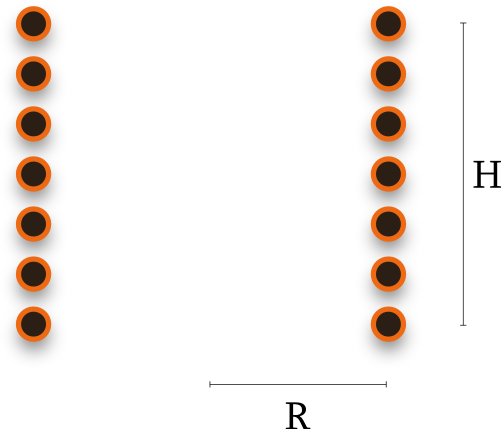Helical geometry.   The inductor looks like a solenoid with widely spaced turns. Such a geometry will cause quite high coupling between the primary and secondary circuits, which is not always a good thing as we discussed in the influence of the coupling subsection. Apart from the overcoupling problem, having a high primary increases the risk of arcing between the top load and the primary, which is potentially catastrophic. Indeed, enormous voltages are applied to a circuit that is not designed to handle them, which can lead to destruction of the primary capacitors or/and the transformer if not properly protected. To solve this issue, the base of the secondary coil can be raised above the primary. This design has not proven very efficient however. If we call R the radius of the helix, H its height (both in centimetres) and N its number of turns, an empirical formula yielding its inductance L in microhenrys is :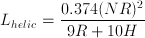Archimedes' spiral geometry.   This geometry naturally leads to a weaker coupling and reduces the risk of arcing in the primary : it is therefore preferred on powerful coils. It is however rather common in lower power coils for its ease of construction. Increasing the coupling is possible by lowering the secondary coil into the primary. Zeus' primary inductor has this shape. Let W be the spiral's width given by W = Rmax - Rmin and R its mean radius, i.e. R = (Rmax + Rmin)/2, both expressed in centimetres. If the coil has N turns, an empirical formula yielding its inductance L in microhenrys is :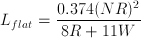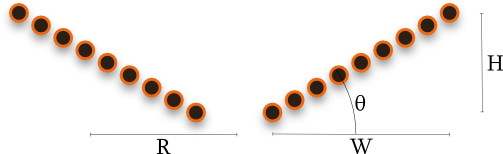Hybrid geometry.   This conical configuration is a sort of compromise. It allow a fair coupling between the two circuits and is rather safe as well. It's the most common configuration for low power coils. A posteriori, I think such a geometry would have been a better choice for Zeus, despite being more difficult to built. I use it on Hyperion with satisfying results. By defining the parameters H, W, R and N as previously, the self-inductance is computed by doing an average of the values Lhelic and Lflat weighted by the angle θ of the cone with the horizontal plane.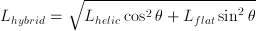#### Case of Zeus

Zeus' primary inductor has a plane spiral geometry, tapped at 8.25 turns. Its internal radius Rmin = 7 cm and its external radius (at 8 turns) Rmax = 44 cm. From here, we find W = 37 cm and R = 25,5 cm following the previous definition. Its computed inductance is then :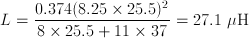which is close to the measured value. It must be kept in mind however that the primary's real inductance will be slightly higher than this value, as the latter neglects the wirings used to connect the components. These wirings indeed form a closed loop which contains some ferromagnetic materials (screws, bolts, etc.). This extra inductance is somewhat wasted as its magnetic field is far from the secondary and will not contribute to it's resonance.

Some details must be taken into account in order to keep this parasitic inductance as low as possible. Avoid loops in the wiring and make it as short as possible. It is also recommended to avoid placing wires parallel to each other to avoid induced currents.

#### Skin effect

To minimize energy losses, the resistance of the primary circuit must be made as low as possible. This implies the use of good conductors as well as a large conduction area. It is easy to imagine the practical limitations of this idea. For example, if we wanted to make a spiral from a 12 meter rod of 1.2 cm diameter made of massive copper (this is roughly the dimension of Zeus' primary inductor). This spiral would weight 12 kg and would require advanced machinery to be bend. And the cost would be terrific.

Fortunately, we can make use of a propriety of high-frequency currents. The so-called skin effect is the fact that, at high frequencies, the current flow mainly on a thin layer near the outer surface of the conductor. (This is not rigorously correct actually. The surface of the conductor on which the current will flow will depend on the ambient magnetic field. For example, in the outer conductor of a coaxial cable, current will flow on its internal surface.) We'll see how this justifies the use of hollow copper tubing (as plumbing pipes), much easier to handle.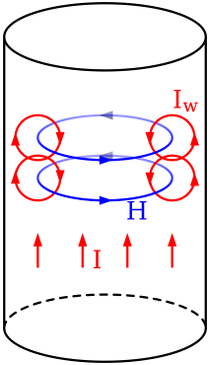Figure 3.18 — Alternating current I creates an alternating magnetic field H, which will generate Foucault currents Iw. [Image credits: Wikipedia].

Skin effect is easily explained by magnetic induction. When an alternating current flows in a conductor, it will generate a magnetic field around it, which is also alternating. Lenz' law then says that these variations of magnetic field will lead to the apparition of an emf that will tend to attenuate these variations. Foucault currents will therefore appear, as shown in fig. 4.18, which will oppose the main current in the centre of the cylinder but not near its edges. When current I goes in the opposite direction, so will H and Iw and the previous discussion holds for both directions.

It can be shown that the current density J(d) in a cylindrical conductor follows an 1/e law from the edges to the centre :

J(d) = JS e-d/δ

where d is the depth (i.e. the distance from the surface), JS the current at the conductor's surface, JS ≡ J(d=0), and δ is the "skin depth", a parameter depending on the material. Taking d=δ in the previous equation shows that δ represents the depth at which the current density is only 0.37 (1/e) times the density in the surface. Computing the skin depth is complicated in the general case. However, with a good conductor and at reasonable frequencies (< 1018 Hz), the following formula is a very good approximation :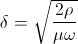where ρ is the resistivity of the conductor (1.7 · 10-8 Ωm for copper), μ is magnetic permeability ( 1.26 · 10-6 H/m for copper), and ω the angular speed of the current, equal to 2πf.

Let's compute the skin depth for copper, the conductor Zeus' primary inductor is made of, at the frequency of 300 kHz, its resonant frequency :

δCu ≈ 0.12 mmFigure 3.19 — Log-log plot of skin depth δ as a function of frequency f for various mediums. [Image credits: Wikipedia].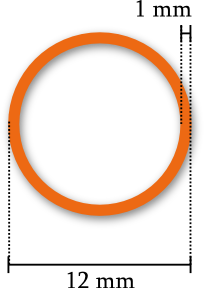We can also calculate the ratio between the current that flows though a plain cylinder and the current that flows into a hollow one. The tubing used for Zeus' winding is 1 mm thick and has a diameter of 12 mm. We find the current I by integrating the density J(r) over the considered cross-section. We set d = R-r, where R is the cylinder's radius (6 mm) and r the radial distance, which we'll integrate. We get :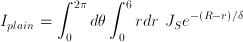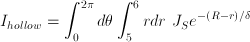Computing these integrals, we find :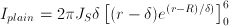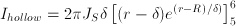We're only looking at the ratio between the two, so the unknown factor JS will cancel out :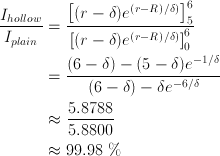In other words, the current gain we'd have by using a plain tube is only 0.02 %, a ridiculous value. Using a hollow tubing is therefore perfectly adapted. The drawback of this high-frequency propriety is that the effective resistance is much higher than in DC, as the current is forced to flow on thin layer instead of on all the conductor (and this, whatever the cross-section of the conductor is).

#### Details on the construction

The primary is supported by a four wooden "arches". I first tried to fix the primary to these supports using epoxy glue but this didn't work because of the spring force of the copper tubing itself. Clearly, the copper tubing had to be deformed into a spiral shape much more than it currently was. I achieved this by screwing the tubing in a spiral configuration on a table. After a while, I removed the screws et recovered the tubing. This way, it would normally have the right shape and no strong force would be required to place in on the arches. In the end, it worked and I could use simple tyraps to fix it.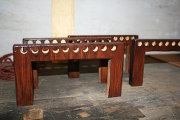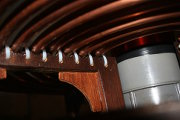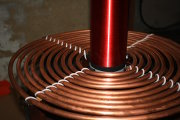Figure 3.20 — Left: The wooden arches, the upper part is actually cut at the hole's half height so the tubing can be placed. Middle and right: Primary coil placed and fixed.

## 3.3   Spark gap

The function of the spark gap is to close the primary LC circuit when the capacitor is sufficiently charged, thus allowing free oscillations inside the circuit. This is a component of prime importance in a Tesla coil because its closing/opening frequency will have a considerable influence on the final output.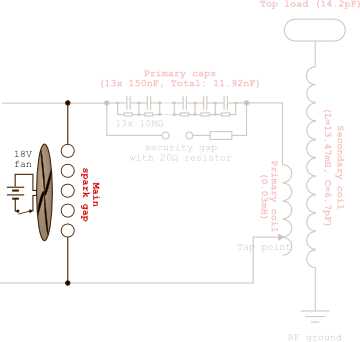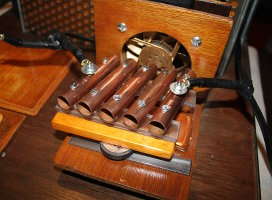Figure 3.21 — Position on schematic (highlighted) and photograph of Zeus' (static) spark gap.

An ideal spark gap must fire just when the voltage across the capacitor is maximal and re-open just when it falls down to zero. But this is of course not the case in a true spark gap, it sometimes does not fire when it should or continues to fire when the voltage has already diminished ; we'll see which factors will make a spark gap efficient.

### 3.3.1   Two types of spark gap

#### Static SG

In a static spark gap, there's two or more electrodes spaced by a given distance. When the potential difference between them rises above some critical value, the air is ionized and thus becomes conducting. This closes this gap, allowing current to flow.

The critical intensity of the electrical field above which air breakdown occurs depends on many parameters (humidity, temperature, air composition, etc) and it is difficult to predict it in the general case ; gas breakdown itself is a very complex phenomenon. The generally admitted value, which is nothing more than an order of magnitude, is EC = 3 · 105 V/m. Using this value in the spark gap design (for example, setting the inter-electrode distance to 1 cm, hoping breakdown will occur when the potential difference between them will reach 30 000 V) will not yield expected results. Indeed, apart from factors related to air itself, the geometry of the electrodes will influence the intensity of the field for a given potential difference as well.

It's much more efficient to built an adjustable spark gap and to find the optimal distance afterwards, by trial-and-error. This is done by first setting the distance to an obviously too short value, lighting the coil and observing the result. Next, spacing the gap a little bit and trying again until the arcs at the top load are the longest. If the electrodes are too close, the gap will fire while the capacitor is not full, which will considerably diminish the coil performances. If they are too distant, the spark will simply not fire and the coil doesn't work. The latter case is much more dangerous as we discussed in the charge at resonance section. If the primary circuit is correctly conceived however, the security gap will take over and will bypass the fragile components. Static spark gaps are easy to construct but generally have poorer performance than their rotating counterpart. They have a tendency to fire erratically and to carry on firing when voltage has diminished. Moreover, the path of ionized air has a certain resistance.

#### Rotative SG

On this kind of spark gap, it's no longer the voltage that closes the circuit. Here, we have basically a conducting rod that spins rapidly between electrodes. When the rod is at its closest distance from the electrodes, current flows via small paths of ionized air. When the rod offsets from this position, current flow stops, which re-opens the gap. There are many ways to build such a rotative gap (several rods, several pairs of electrodes, synchronous or asynchronous motor, etc.) but the subject won't be covered here. This kind of spark gap is more precise and yields better results, but is more difficult to construct and adjust, the main difficulty being that the rod must pass in front of the electrodes just when the voltage difference across the capacitor is at its maximum.

Zeus' spark gap is a static one. As mentioned earlier, they tend to remain open after the voltage has diminished. This happens because the path of ionized air demands a much lower voltage to live on than it requires to be created.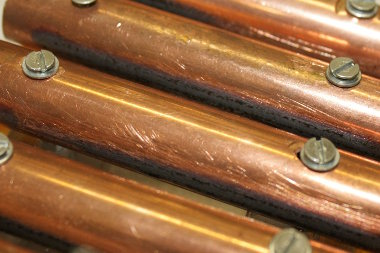Figure 3.22 — Heavy corrosion between the copper tubes.

In order to improve its performances, the gap has to be quenched, that means to be forced open. Here's a few strategies for that. One is to force a large, continuous flow of air inside the spark gap, in order to chase the ionized air. Sucking the air is also possible (for example, with vacuum cleaner parts) and gives good results as well. Blowing air into the gap has many benefits. This not only quenches the gap but also cools it. Because the critical breakdown field decreases with temperature and the high current crossing the spark gap will indeed increases its temperature, the spark gap will fire at a lower voltage after a few seconds (and the sound of the spark gap becomes notably more high-pitched), hindering the coils performances. Thus cooling the gap will prevent such problems. It is also possible to use more than two electrodes. The single arc will then be divided into so many smaller arcs, which will quench more easily. The drawback of this configuration is that all these sub-arcs will act like series resistors : all of them will dissipate energy in the form of light, heat and noise. The Richard-Quick design makes use of several copper tubes disposed in parallel. I used this model for Zeus' gap.

The spark gap has to deal with enormous current, with transient spikes of nearly 300 amperes. This leads to the apparition of strong corrosion on the electrodes, which will also hinder the coils performances. It is necessary to regularly clean/sand them.

1st version.   The first spark gap I built was made of 5 identical copper tubes, 10mm in diameter, 80mm long. These were fixed on a flexible membrane following a design found here. By bending this membrane, one can modify the distance between the electrodes. The whole assembly was placed in front of a 12 V personal computer fan with a diameter of 80 mm. Power was supplied by two 9 V batteries connected in series.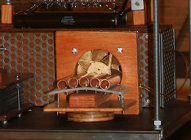Figure 3.23 — v.1 mounted on Zeus' chassis.

The membrane must be made of an insulting, fire-resistant material and I used foamed PVC, which is at least auto-extinguishable. Its flexibility was not excellent, however, and didn't allow for a good distance setting. As I tried to bend it more, it eventually broke. This spark gap was not easy to maintain either. The repeated action of sanding the surfaces had a tendency to loosen the fixations of the tubes. A better design was thus to be found.

2nd version.   This spark gap is made of the same copper tubes as before. The difference here is the mounting. The tubes are loosely fixed at both extremities on PVC supports. The side close to the fan is immobile while the other side can be horizontally translated (the magnet on the metal bar ensures the stability of the assembly). The spacing between the tube can thus be adjusted with a shearing movement. This design proved more precise and easier to maintain.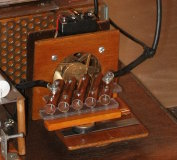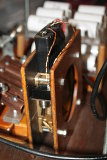Figure 3.24 — Left: v.2 mounted on Zeus' chassis. Right: The power supply of the fan.

You may also want to have a look at the improved spark gap design installed on my second coil.

## 3.4   Secondary circuit

### 3.4.1   Coil

The function of the secondary coil is to bring an inductive component to the secondary LC circuit and to collect the energy of the primary coil. This inductor is an air-cored solenoid, generally having between 800 and 1500 closely wound adjacent turns. In the Zeus Tesla coil the secondary coil is made of enamelled copper "magnet wire" with a diameter of 0.5mm (SWG 24) which is wound around a PVC pipe of 100mm external diameter. The coil is 40.2 cm tall.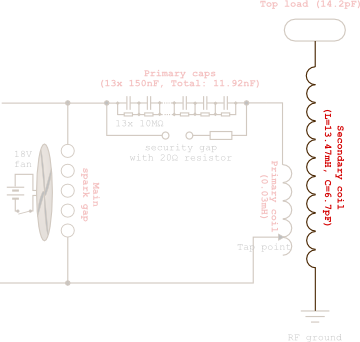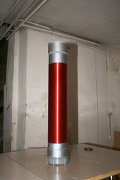Figure 3.25 — Position on schematic (highlighted) and photograph of Zeus' secondary coil.

To calculate the number of turns that have been wound, this quick formula will avoid a certain fastidious work:

N = H/d

where H is the height of the coil and d the diameter of the wire used. In our case, the number of turns is 790 turns (more or less a few turns). It is quite low, but the diameter of the coil is rather large given the relatively small power of the coil ; this will provide a fair self-inductance while limiting parasitic capacity. Another important parameter is the length l we need to make the entire coil. It is given by

l = 2πNr

where r is the radius of the coil. Zeus' coil has thus necessitated 248 meters of wire. One can show that the self-inductance L in henrys of a adjacent-turns solenoid is given by

L = μN2A/H

where μ represents the magnetic permeability of the medium (≈ 1.257 · 10-6 N/A2 for air, very close to the value of the void), N the number of turns of the solenoid, H its total height, and A the area of a turn. Injecting Zeus' coil values, we get

Lth = 15.17 mH

which is not too far from the value measured from an LCR bridge :

Lexp = (11.92 ± 0.01) mH

#### Characteristics

Resistance.   During the first tries of Zeus, I had placed the secondary coil lower in the primary spiral in order to increase coupling. This didn't yield the expected results : arcing between the primary and secondary occurred, which damaged the coil at several points, as shown in Fig. 4.29. I thus put the secondary coil back in its initial position. Fortunately, the coil hasn't been sectioned. The only noticeable consequence was an increase of the coil's resistance, increasing from 20 to 25 Ohms.

However, this value was measured with a classical ohmmeter, i.e. with a direct current. But what will flow in the coil is high-frequency alternating current. A more precise measure would include effects such as skin effect and proximity effect. The method proposed by Fraga, Prados and Chen takes these effects into account and has been proven to be precise for solenoids (see JAVATC page on the subject). The simulator yielded the following value :

Rac = 94.856 Ω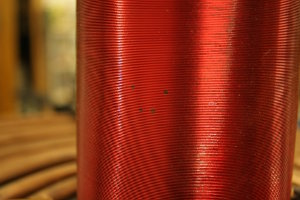Figure 3.26 — Results of inadequate space between the coils : arcing between the two occurred, burning the secondary coil at several points.

Inductance and capacitance at resonance.   The inductance calculated and measured previously are again valid for low frequencies only. The self inductance of the secondary coil driven at resonance is slightly different, because of the non-uniform repartition of current and because the length of the coil is comparable to the wavelength of the signal it will carry. Once again, a more precise formula must be used (see JAVATC page on the subject). The simulator gave the following value :

Lres = 13.471 mH

It's also interesting to know the (parasitic) capacitance of the coil. Here also the formula is complicated in the general case (see JAVATC page on the subject). We'll use the value yielded by JAVATC :

Cres = 6.74 pF

Q factor.   We can now compute the Q factor of the coil, following formula established in the last chapter, Q = R-1(L/C)1/2. To get a coherent result, we'll use the previous values for R, L and C (with top load capacitance added). We thus get :

Q = 285

Which is a rather good value, that will indicate low losses in the secondary LC circuit. It is difficult to surpass 500 with common conductors. Note that it is also possible to measure the Q factor experimentally following the procedure detailed in JAVATC, Q factor page.

#### Quarter-wave antenna

We saw in the quarter-wave antenna subsection of the theory chapter how we could apply this model to the Tesla coil. In the next section, we'll calculate the resonant frequency f of the secondary coil to be 3.19 · 105 Hz. The corresponding wavelength is λ = c/f (where c is the speed of propagation of the wave : here, c ≈ 2.998 · 108 m/s), i.e. approximately 940 m. In other words,

λ/4 = 235 m

As the total length of the wire in Zeus' secondary coil is around 248 meters, we can conclude it is correctly tuned like a quarter-wave antenna. But as mentioned earlier, this is not necessary , that is why we performed such rough calculations. (Actually, we should have used the speed of propagation of the wave in copper for c, instead of the vacuum, but both value are actually rather close.)

#### Construction

This component is not especially difficult to build but requires a lot of patience. The 800 turns have been wound entirely by hand and took a few days. Precautions had to be taken in order to prevent sweat from infiltrating the coil. The very high voltages involved can cause arcing between turns if the coupling is set too high (see Influence of coupling, page 2). It is common to apply an insulating resin on the coil, which will reinforce the insulation already provided by the polyurethane covering the magnet wire. I couldn't find such a resin so I instead applied liberal amounts of insulating spray (the same used in the capacitors).

There's an important empirical rule about the ratio between the height H and the diameter D of the coil. It has been observed that the best performances are attained with an H/D ratio between 3 and 5. Zeus' coil is thus conform with a ratio of 4.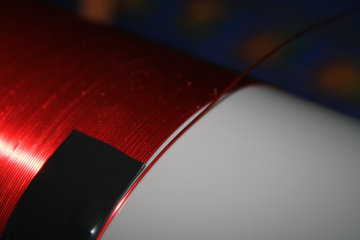Figure 3.27 — "Keep calm and carry on."

The top load acts like the upper "plate" of the capacitor formed by the top load and the ground (see Distribution of capacitance within the secondary circuit, page 3). It adds capacity to the secondary LC circuit and offers a surface from which arcs can form. It is possible, actually, to run a Tesla coil without a top load, but performances in terms of arc length are often poor, as most of the energy is dissipated between the secondary coil turns instead of feeding the sparks. The are many different possibilities regarding the shape of the top load, the most common ones being the sphere and the toroid. Computing its capacitance is difficult in the general case. A collection of empirical formulas are given in K. Wilson's page on top load design. The JAVATC program gave the next value :

Cs = 14.172 pF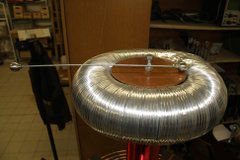Figure 3.28 — Position on schematic (highlighted) and photograph of Zeus' top load.

#### Influence of geometry

The top load is perhaps the most important component when determining the final arc length (except the transformer of course). There is a lot of discussion about its optimal size. In a general way, a larger top load will have a larger capacitance. Regarding the formula specifying the voltage gain (established page 3), one could think it is desirable to keep the secondary capacitance as low as possible, as Vout decreases when Cs increases. However, if the top load capacitance is low (or non-existent), the majority (resp. the totality) of the secondary capacitance will be located in the secondary coil itself. Then, the energy of the secondary circuit is mainly dissipated as heat between the turns instead of feeding the sparks. Hence an optimal capacitance exists.

The toroidal geometry is often preferred instead of the spherical. If the top load is a sphere, the near electrical field will be more uniform, which will result in more numerous, shorter sparks. But with a toroidal geometry, the electrical field is more intense on the outer edge of the toroid : there will be fewer sparks but longer on average, most of the arising form this outer edge.

Be it toroidal of spheric, the curvature radius of the load will increase with its size. And the larger the curvature radius is in a certain zone, the weaker the electric field will be. Fewer spark will be able to form, and the available energy for each of them will be greater. This will result in less numerous but longer sparks. It is even possible to make the top load big (and smooth) enough so not a single arc can form. Conversely, if the curvature radius is smaller, the electrical field will be intense in many zones, allowing more sparks to be formed ; these will be shorter as the available energy will have to be distributed between all these arcs. There is thus also an optimal size to the top load.

State of the surface.   Another important parameter influencing the final arc length is the roughness of the top load surface. If the surface has asperities, the electric field near these will be much more intense. It can indeed be shown from Maxwell's equations (Gauss' law to be precise) that the electrical field is more intense near zones of strong curvature. This fact is called the spike effect.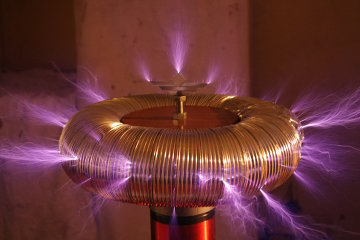Figure 3.29 — Numerous sparks arising from the top load asperities. Notice Zeus' Eagle. 1s shutter speed.

If one wishes to get longer sparks, it will be judicious to have a smooth top load ; to reduce the number of breakout points and thus of sparks (this worked well for Hyperion's smooth toroid).

Addition of a breaking point.   In order to force sparks to be generated at a single point (and thus having longer arcs), it is possible to add a breaking point to the top load. By spike effect, this will ensure the electrical field is comparatively much stronger near this zone. It has been suggested that the breakout point should be a ball instead of a spike. Indeed, because of the higher temperature of the ionized air path, the arc will have a tendency to rise, and a little sphere would allow the spark base to "glide" on it as it moves, giving more time for the spark to develop (see Description of a cycle, page 2).Figure 3.30 — Adding a spike forces the sparks to break from a single point, to a certain extent. Their length also increases. 3.2s shutter speed.

#### Details of construction.A toroidal top load was used on Zeus. There are a few empirical rules regarding the dimension of the toroid. Let's call d its minor diameter and D its major diameter, like shown in adjacent figure. d should be equal to the secondary coil diameter (10 cm here) and the D/d ratio should be between 3 and 4. The top load position is also subject to some guidelines. If it is too close from the top of the secondary coil, its electrical field will "drown" the last spires, which will result in a lower final voltage. Conversely, if the top load is too high, its electrical field cannot protect the last spires and the risk of inter-arc spark and Corona losses increases. If we call h the distance between the base of the top load and the top of the secondary coil, it is recommended to have dh.

1st version.   The first toroid I made had a minor diameter d = 8 cm and a major diameter D = 28 cm. Its theoretical capacitance was 13.5 pF. It was made of flexible aluminium duct. It has been rolled around a support made of two discs, one in wood (top) and the other in aluminium (bottom). The toroid was closed with aluminium tape. The connection with the secondary coil was made with a small block connector. On the next picture, one can spot a small Corona "leak" at this point because of the roughness of the screws. This toroid produced the very first sparks of Zeus. Their size was modest, because of the capacity of the top load was too low. To enhance performances, K. Wilson suggested to build a new, larger toroid.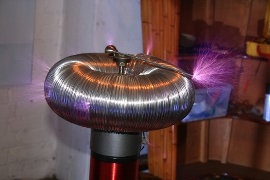Figure 3.31 — The very first sparks of Zeus. A lot of emotion. 1s shutter speed.

2nd version.   This was the final version of Zeus' toroid. The same construction method was used, only dimensions changed : d = 10 cm, D = 35 cm. The rod serving as a breakout point is attached with a neodymium magnet. Fig. 3.30 attests of the great difference between the two top loads in terms of arc length. I just had to retune the primary inductance in order to have the two circuits resonate. We'll discuss this point in the next section.

## 3.5   Resonance tuning

Setting the primary and secondary circuits at resonance, i.e. have them share the same resonant frequency, is of prime importance for good operation. As discussed in the section about RLC circuits, the response of an RLC circuit is the strongest when driven at its resonant frequency. In a good RLC circuit, the response intensity falls sharply when the driving frequency drifts from the resonant value.

Let's have a look at Zeus' case. We compute the secondary resonant frequency with its capacitance Cs and its inductance Ls with the well-know formula f = 1/(2π(LsCs)1/2). The secondary capacitance is equal to the coil capacitance plus the top load capacitance, computed by taking into account the non-uniformity of the current distribution. But the non-uniformity of the current distribution. The JAVATC simulator gives a value for the total secondary capacitance (Effective shunt capacitance), Cs = 18.425 pF. Let's also recall the secondary inductance : Ls = 13.471 mH. We thus get

fres = 319.46 kHz

### 3.5.1   Tuning methods

The tuning is generally done by adjusting the primary inductance, simply because it's the easiest component to modify. As this inductors has wide turns, it is easy to modify its self-inductance by tapping the final connector at a certain place in the spiral. The simplest method to achieve this adjustment is by trial-and-error. For this, one begins to tap the primary at a point supposedly close to the resonant one, lights the coil and evaluates arc length. Then the spiral is tapped a quarter of turn forward/backward and one re-evaluates the result. After a few tries one can proceed with smaller steps, and will finally get the tapping point where the arc length is the highest. Normally, this tapping point will indeed set the primary inductance such as both the circuits are at resonance. I used this procedure for Zeus and tapped the primary to 8.25 turns.

This method isn't infallible however. It presupposes that there is one and only one resonant point. This would be true if current and voltage distribution were uniform. And we saw the secondary coil has a distributed capacitance and deals with non-uniform current/voltage distributions. This may lead to the apparition of several secondary resonances (see Fig. 5.12), i.e. local but not necessarily global impedance minima. By proceeding though the trial-and-error method discussed above, one can think they've reached the true resonant value but actually have a secondary resonance.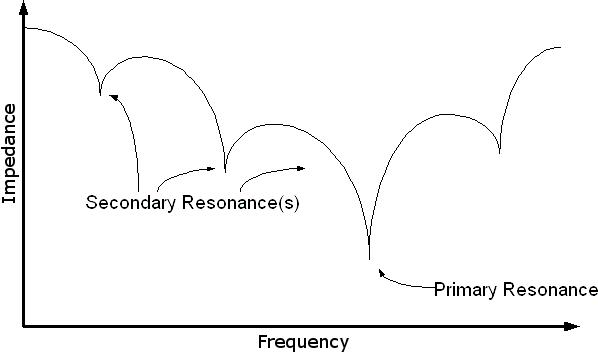Figure 3.32 — Because of the non-ideal behaviour of the components, the secondary circuit can exhibit more than one impedance minimum.
[Image credits: Jamie Oliver]

A more precise method would involve an analysis of the individual response of both the circuits (in the coupled configuration, of course, i.e. without physically separating the circuits) with a signal generator and an oscilloscope. Detailed procedures are given in J. Oliver's Tune a coil and P. Tuck's Oscilloscope tuning webpages. Note that arcs themselves can produce some extra capacitance, it is therefore advised to set the primary resonant frequency slightly lower than the secondary, in order to compensate for this effect. However, this is noticeable only with very powerful coils (which can produce arcs longer than 1m), and should have little influence on Zeus.

## 3.6   RF ground

It is the ground at which the bottom of the secondary coil is connected. Its role is to keep the voltage at the base of the coil at zero, but also (more importantly) to act like the second "plate" of the top load-ground capacitor. In this light, it makes the secondary capacitance less dependent of the immediate environment.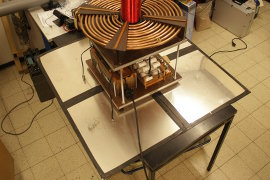Figure 3.33 — Zeus' RF ground is made of an 1 squared meter aluminium plate.

The best grounding method is to use a metallic rod stuck into the soil ; when this option is not possible, one has to make his own ground. For this purpose, it is possible to use a large metallic plate, placed underneath the Tesla coil. Its total width should be equal to twice the distance between the top load and the base of the secondary coil. Zeus being 1.10m high, a plate 2.2m wide would be required. The plate used on Zeus is only 1m wide but seems to be sufficient. It is made of 4 panels of 50cm × 50cm bound with aluminium tape and covered by stronger, convectional tape for protection. This allow the plate to be folded, which increases transportability. Zeus' plate is made of plain metal, but it is also possible to use a conducting mesh. This is actually better as it prevents some Foucault currents from taking place.

## 3.7   Other components

Finally, let's discuss the "optional" components a Tesla coil can have. A Tesla coil can work without, but their presence is nevertheless recommended for security- or performance-related issues.

### 3.7.1   NST protection filter

Tesla coils give a harsh treatment to the main transformer. We saw that it must withstand powerful transient currents and voltages as well as high-frequency alternating currents. To ensure long transformer life, it is highly recommended to protect it. We mentioned earlier that it's not possible to use a differential circuit breaker, as it will open the circuit as soon as resonant amplification begins to take place, thus forbidding any Tesla coil use. T. Fritz has however designed an NST protection filter specifically made for Tesla coil operation (the "Terry filter"). It is made of a grounded spark gap, low-pass filters and varistors, and is to be connected between the transformer and the primary circuit. Such a filter has been used on Zeus.

Note that a filter is necessary only with NSTs, because of their fragility. Other kinds of transformers (MOT, pole pigs, etc) are rugged enough to support Tesla coil operation as is.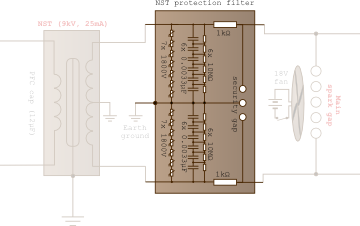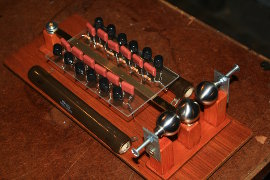Figure 3.34 — Position on schematic (highlighted) and photograph of Zeus' NST protection filter (wires not yet present).

#### Spark gap

It is made of three electrode (or four, a pair per side), the central one being connected to ground. If a voltage spike occurs, the gap fires and the dangerous current is redirected to ground, thus sparing the transformer.

#### RC low-pass filter

It is physically just behind the spark gap and is made of a 1000 Ω, 100 W ceramic resistor (datasheet) and of 6 high-voltage capacitors of 3.3 nF each (datasheet), thus totalising 0.55 nF. Here also, bleeder resistor were soldered in parallel of each capacitor (the same ones used in the primary capacitor, see here). Initially, there was an arcing problem between these resistors and the wooden support on which they lied, but a PVC support solved the issue.

The resistors cause very little energy loss as the current is very weak (25 mA rms). The average loss P is given by P = RI2 = 0.25 W, which is really negligible in comparison of the 225 watts supplied. Note also that they help preventing the current flowing into the primary to come back into the transformer and beyond. Indeed, the impedance of the main spark gap firing is negligible in front of the total impedance of the NST and the filter : when it fires, it almost short-circuit these components and the high primary currents stay where they are. In sinusoidal regime, the cutoff frequency of a low-pass filter is the frequency above which the transmitted intensity will be less than 0.707 (which is the square root of 1/2) times the input intensity. One can show it is given by:

fc = 1/(2πRC)

We thus find the cut-off frequency to be around 287 kHz. The filter will therefore further help block the primary currents (around 320 kHz for Zeus), but more importantly, they will block the interferences of much higher frequencies. For more information, refer to R. Burnett's page on frequency splitting.

#### Varistors

Metal oxide varistors (MOVs) complete the protection filter. These non-linear components have their resistance drop dramatically above a certain voltage. Here, this threshold is 1800 V in direct current and 1000 V in alternating current (datasheet). As there are 7 of them on each side, the total threshold voltage is 7000 V. The voltage difference between a wire and the ground is 4500 V rms, so the amplitude is 6364 V, which is just below the threshold. These components help to protect the NST from voltage spikes in case the spark gaps don't fire. Actually, they're not strictly necessary but they undoubtedly add some protection.

#### Ground

The central wire of this filter should not be connected to the safety ground. Voltage and current spikes as well as high-frequency interference would be sent, which is hazardous for electronic apparatuses connected to the mains. A proper ground for this filter is the soil.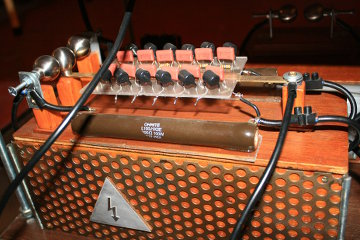Figure 3.35 — The protection filter assembled. The NST is in the wooden box just under the filter.

### 3.7.2   PFC capacitor

The power average over a period T of an alternating current is defined as following :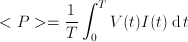where current and voltage are definition by sinusoidal functions of same frequency, but whose amplitude and phase can change. One can easily prove the next result :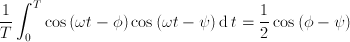We thus see that the power is maximum when voltage and current are in phase.

The high self-inductance of the transformer causes the current to lag the voltage, which results in less transmitted power as we just saw. To overcome this problem, i.e. re-phase both signal, one connects a capacitor in parallel to the transformer inputs. In a capacitor, it is indeed the voltage that is delayed with respect to current. This is the role of the power factor correction capacitor (PFC cap).Figure 3.36 — Position on schematic (highlighted) and photograph of Zeus' PFC cap.

This capacitor must be able to work continuously, i.e. must be a run-type capacitor. The start-type capacitors on the other side are designed to work only during short time intervals. One can show the ideal capacitance of the PFC cap is given by :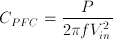where P is the transformer power, f is the mains frequency (50 Hz in Europe), Vin is the mains voltage (230 V). This yiels a value of 13.54 μF for Zeus. The capacitor used is 12 μF (see datasheet). Having the exact value doesn't matter as long as it is "close enough".

### 3.7.3   AC line filter

In order to protect the electronic devices connected to the mains from dangerous transient currents/voltages and from radio interferences generated by the Tesla coil circuits, it is recommended to connect a last filter between the mains and the transformer : an EMI suppressor, or line filter.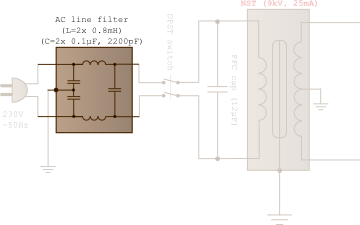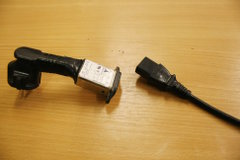Figure 3.37 — Position on schematic (highlighted) and photograph of Zeus' AC line filter.

Those interferences are normally blocked by the NST protection filter, but it is perhaps not a good idea to fully rely on a DIY component to protect the home electrical installation. It is advised to place it as far as possible from the Tesla coil to avoid the apparition of induced current above the filter, hence reducing its effectiveness. Note that some people advise to connect it upside-down (with "load" being the line and vice-versa).

That's it for the construction as well ! which terminates this document. For anyone interested, the JAVATC output for Zeus, which contain all the dimensions and characteristics of this coil, can be downloaded from the link on the right.